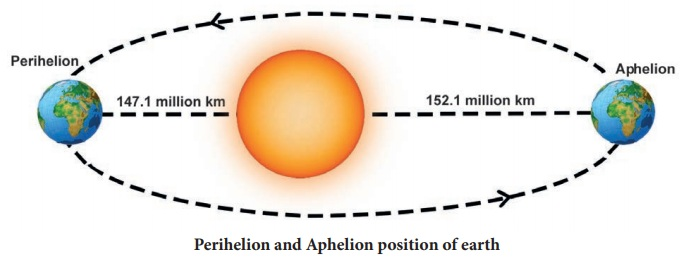Home | | Science 7th Std | Measuring distance of celestial bodies

# Measuring distance of celestial bodies

Normally, we use centimeter, metre and kilo metre to express the distances that we measure in our day to day life.

Measuring distance of celestial bodies

Normally, we use centimeter, metre and kilo metre to express the distances that we measure in our day to day life. But, for space research, astronomers need to measure very long distances such as the distance between the earth and a star or the distance between two stars. To express these distances, we shall learn about two such units, namely,

i. Astronomical unit

ii. Light year

Water has more density thanoils like cooking oil and castoroil, although these oils appear to be denser than water. Density of castor oil is 961 kg/m3. If we put one drop of water in oil, water drop sinks. But, if we put one drop of oil in water, oil floats and forms a layer on water surface. However, some oils are denser than water.

Astronomical unit

We all know that the earth revolves around the sun in an elliptical orbit. Hence, the distance between the sun and the earth varies every day. When the earth is in its perihelion position (Perihelion is position of the shortest distance between the earth and the sun), the distance between the earth and the sun is about 147.1 million kilometre. When the earth is in its farthest position, that is when the distance between Earth and Sun is the largest (called aphelion position) the distance is 152.1 million kilometer. The average distance between the earth and the sun is about 149.6 million kilometer. This average distance is taken as one astronomical unit.

Neptune is 30 AU away from the Sun. It means it is thirty times farther than the Earth.

One astronomical unit is defined as the average distance between the earth and the sun.

1 AU = 149.6 million km = 149.6 × 106 km = 1.496 × 1011 m.

Light year

The nearest star to our solar system is Proxima Centauri. It is at a distance of 2,68,770 AU. We can clearly see that using the AU for measuring distances of stars would be unwieldy. Therefore, astronomers use a special unit, called ‘light year’, for measuring the distance in deep space. We have learnt that the speed of light in vacuum is 3 × 108 m/s. This means that light travels a distance of 3 × 108 m in one second. In a year (non-leap), there are 365 days. Each day has 24 hours; Each hour has 60 minutes; Each minute has 60 seconds.

Thus, the total number of seconds in one year = 365 × 24 × 60 × 60

= 3.153 × 107 secondIf light travels a distance of 3 × 108 m in one second, then the distance travelled by light in one year = 3 × 108 × 3.153 × 107 = 9.46 × 1015 m. This distance is known as one light year.

One light year is defined as the distance travelled by light in vacuum during the period of one year.

1 Light year = 9.46 × 1015 m.

In terms of light year, Proxima Centauri is at 4.22 light-years from Earth and the Solar System (and Earth). The Earth is located about 25,000 light-years away from the galactic center.

Tags : Measurement | Term 1 Unit 1 | 7th Science , 7th Science : Term 1 Unit 1 : Measurement
Study Material, Lecturing Notes, Assignment, Reference, Wiki description explanation, brief detail
7th Science : Term 1 Unit 1 : Measurement : Measuring distance of celestial bodies | Measurement | Term 1 Unit 1 | 7th Science